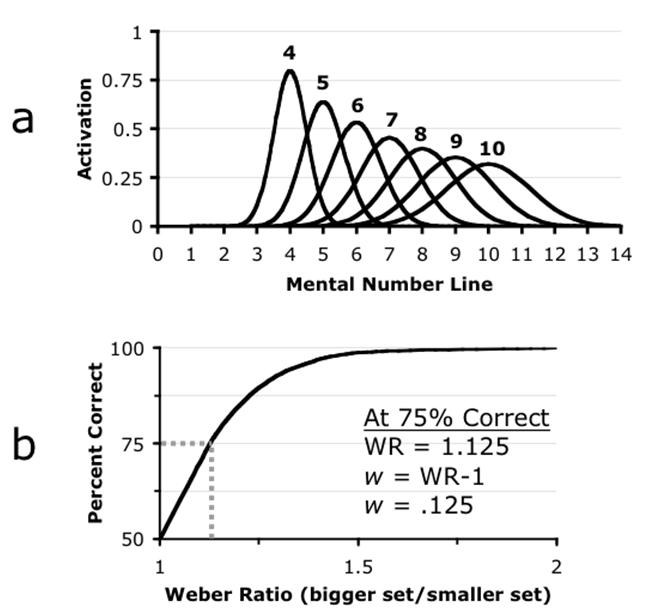# What is a Weber Fraction?

## Model Representations of the ANS

In modeling performance on tasks that engage the ANS, it is necessary first to specify a model for the underlying approximate number representations. It is generally agreed that each numerosity is mentally represented by a distribution of activation on an internal “number line.” These distributions are inherently “noisy” and do not represent number exactly or discretely . This means that there is some error each time they represent a number; and this error can be thought of as a spread of activation around the number being represented.## The Mental Number Line

The mental number line is often modeled as having linearly increasing means and linearly increasing standard deviation . In such a format, the representation for e.g., cardinality seven is a probability density function that has its mean at 7 on the mental number line and a smooth degradation to either side of 7 such that 6 and 8 on the mental number line are also highly activated by instances of seven in the world. In Figure 1a I have drawn idealized curves which represent the ANS representations for numerosities 4-10 for an individual with Weber fraction = .125. You can think of these curves as representing the amount of activity generated in the mind by a particular array of items in the world with a different bump for each numerosity you might experience (e.g., 4 balls, 5 houses, 6 blue dots, etc). Rather than activating a single discrete value (e.g., 6) the curves are meant to indicate that a range of activity is present each time an array of (e.g., 6) items is presented . That is, an array of e.g., six items will greatly activate the ANS numerosity representation of 6, but because these representations are noisy this array will also activate representations of 5 and 7 etc with the amount of activation centered on 6 and gradually decreasing to either side of 6.